# GRE Subject Test: Math : Lagrange's Theorem

## Example Questions

### Example Question #321 : Series In Calculus

Let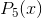be the fifth-degree Taylor polynomial approximation for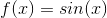, centered at.

What is the Lagrange error of the polynomial approximation to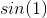?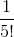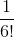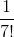Explanation:

The fifth degree Taylor polynomial approximating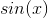centered atis: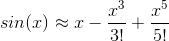The Lagrange error is the absolute value of the next term in the sequence, which is equal to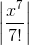.

We need only evaluate this atand thus we obtain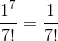### Example Question #1 : Alternating Series With Error Bound

Which of the following series does not converge?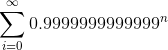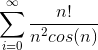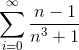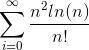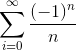Explanation:

We can show that the seriesdiverges using the ratio test.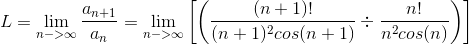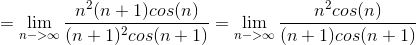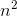will dominate over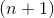since it's a higher order term. Clearly, L will not be less than, which is necessary for absolute convergence.

Alternatively, it's clear that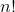is much greater than, and thus havingin the numerator will make the series diverge by the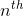limit test (since the terms clearly don't converge to zero).

The other series will converge by alternating series test, ratio test, geometric series, and comparison tests.

### Example Question #1 : Applications Of Partial Derivatives

Find the minimum and maximum of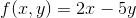, subject to the constraint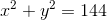.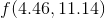is a maximum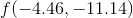is a minimum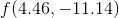is a maximum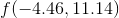is a minimumis a maximumis a minimumis a maximumis a minimum

There are no maximums or minimumsis a maximumis a minimum

Explanation:

First we need to set up our system of equations.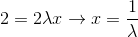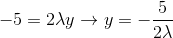Now lets plug in these constraints.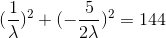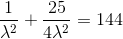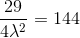Now we solve for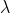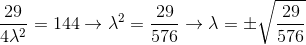If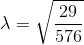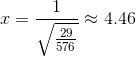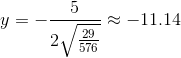If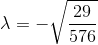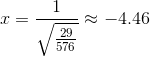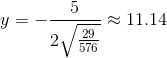Now lets plug in these values of, andinto the original equation.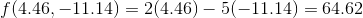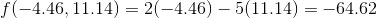We can conclude from this thatis a maximum, andis a minimum.

### Example Question #1 : Lagrange Multipliers

Find the absolute minimum value of the function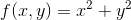subject to the constraint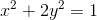.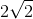Explanation:

Let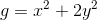To find the absolute minimum value, we must solve the system of equations given by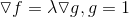.

So this system of equations is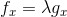,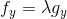,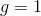.

Taking partial derivatives and substituting as indicated, this becomes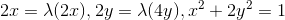.

From the left equation, we see eitheror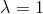. If, then substituting this into the other equations, we can solve for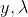, and get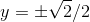,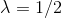, giving two extreme candidate points at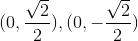.

On the other hand, if instead, this forces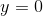from the 2nd equation, and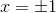from the 3rd equation. This gives us two more extreme candidate points;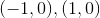.

Taking all four of our found points, and plugging them back into, we have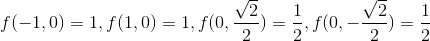.

Hence the absolute minimum value is.

### All GRE Subject Test: Math Resources